# A student project course page 1 of 3

Status
Not open for further replies.

#### hobbyist

Joined Aug 10, 2008
892
This course outline is for the student to take the mundane learning from the books about transistor class of operations, and RC networks, impedance matching, and capacitive coupling, to a robust hands on, logic thinking purposefully design of system and subsystems at the component level, to achieve results that are only read about in the books, where they learn hands on about the pitfall in designing stages and coupling them together, and how to work around those pitfalls by using the book knowledge of how components work together to actually putting them together to get desired results.

As the saying goes, "necessity is the mother of all inventions",

So it is in this, when you find it necessary to come up with a network to eliminate distorting a signal feeding into it, you grab a hold of that book page about impedance matching and finally low impedance and high impedance stuff you read about starts to make sense, OH now I see what they mean about making the input impedance higher than the signal source output impedance, it clicks in now.

So this is what they mean about how an emitter follower is used for impedance matching a high impedance to a low impedance output. It makes sense now. When you are faced with design challenges hands on, thats when all those things you read about starts to ring a bell.

You always wondered "why you need to learn about these transistor amp. class of operations, big deal," yeh, well when your faced with a design challenge of what works best for this application at this stage of design, youll be thankfull you learned about those diff. class of operations. Youll appreciate how you learned about analog and switching designs for transistors.

And those boring RC networks you read about, again when you need a specific timing signal to make something work properly, youll appreciate learning what a RC netwoprk is good for.

This course is to spark interest in the mundane book learning, of circuit design, by giving an oppurtunity for the student to get right down, past those little black boxes and to get into the component level itself, and to really appreciate the book learning at a hands on level.
While others are using arduinos, and micro controlers to build a servo driver, you can impress your professor, by showing how you can design a servo motor driver at the transistor level.

By the time you finish this course, you should be able to know exactly how to design each stage of this driver, using design formulas, and logic thinking, you should also be able to predict what your signals should look like on a oscilloscope, at each stage of the design, and why the signals look the way they do, and for what purpose they are designed to do.

Then if you want to get creative, for extra credit, you can logically design an input circuit that uses two push button switchs to make the servo turn left and right, as long as the switchs are active. (That requires aquiring a knowledge of capacitor timing circuits) You can also design light detector circuits to add on to this, the possibiities are up to how well you can first understand this basic system of circuits.

Lets begin:

An oscilloscope is definately needed.

You can purchase for under \$100 those PC scopes, any kind of a DSO will do, analog scope will work to, it just will be more challenging to watch the waveforms at the low frequencies required.

First purchase a small servo motor, and usually it will show the driver specifications to make the motor work properly. The motor I have specs out as,(PWM period 20mS. with 1-2mS. duty cycle) (4-6 volts).
Ok what does that mean, the motor is looking for a pulse between 1-2mS, every 20mS, in order for it to run, the 20mS is equal to (50Hz, as you take the inverse of 20mS).

So in order to get this kind of a waveform we need to design a PWM circuit. pic.1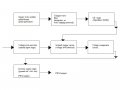Those stage terms are my own, lables.
So here is what were designing, we start out by designing a pulse generator, to satisfy the motor specs as far as the period and pulse width needed to drive the motor circuit.
The pulse generator is designed to have a 2mS pulse every 20mS. since the maximum pulse width is given in the specs to be around 2mS. The exact values are not going to be attained due to component variations without any tweaking, however if we get our values close enough the motor circuit will still work fine enough, for our purposes.

NOTE:
I am designing this as I go along, so I can verify this will be a working circuit when this course is finished.
-----------------------------------------------------------------------------------------------------------------

Schematic shown below,

Here are the design formulas for designing a pulse generator:

I need a pulse generator to produce a 2mS pulse every 20mS.
T=period of cycle. (20mS)
t2= +pulse =(2mS)
t1= negative pulse = (T - t2)= (20mS - 2mS)= 18mS
The output pulse transistor is Q2.
When Q1 is at cutoff, then its collector goes high, thransistor Q2 switchs on and the output is a negative pulse.
Q1 is turned off during the charging up of the cap. C2 through R3.
The design formulas for determining the base resistor values is as such:

first choose a value for the capacitors, I chose 1uF, you can choose any value you want within reason of the frequency required.
Now use this formula to solve for a resistor value:

R=(t / (0.69 * C))
In the example shown:

R3 = (t1 / (0.69 * C2)) therefor R3 = ( 18mS / (0.69 * 1uF)) ~= 26086 ohms, choose 27K ohms.
R4 = (t2 / (0.69 * C1)) which is R4 =(2mS / (0.69 * 1uF)) ~= 2898 choose 2.7K ohms.
R1 = (R3 / 10) = 2.7K ohms
R2 = R2 =(R4 / 5)= 560 ohms

The diode arrangement is used to square up the output waveform. R2's values are the parallel combination of (R4 / 10) .
always choose the closest resistor values available.
Now this should run at a Vcc value from 4 - 12 volts DC.

build this and using an oscilloscope, check to see how close you have a positive pulse of around 2mS wide, every 20mS.
You should be close enough to make it work, if not, than always tweak values to get your optimum desired performance. pic.2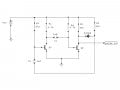pic.3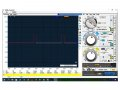Again you may have to tweak the values to get an appropriate signal output.

Here I interject, study the schematic, and use hands on approach to experiment, with this circuit building block, until you are able to use the design formulas given above to design any type of pulse generator, with any kind of period and pulse width.
Once you are satisfied with that, then it's time to move on to the next stage.

Alright the next stage is going to be an RC network, it's actually a low pass filter, however it is used to modify the pulse wave into a ramp wave, so as to produce a sort of a wedge signal.

the design goes as follows:

we need to make this stage with an input impedance high enough to have very little affect on the pulse generator stage that feeds into it. so lets investigate the pulse generator output impedance for a moment.

we have two 560 ohm resistors in parrallel which is around 280 ohms. A good rule of thumb is to make the impedance that a source is working into to be around 10 times or more larger than the impedance of the signal source itself.

so 10 times 280 ohms would be 2.8K ohms. I'll choose a 3K ohm resistor. (closest standard value)
This will be the input impedance (Zin) looking into the next network were going to design.

So this next stage is an RC filter, (waveshaping network, ramp wave, integrator). it looks like this: pic.4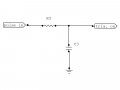R6 and C3 form the RC lowpass filter, that is designed to work as an integrator, to produce a ramp wave.
We already established the Zin should use a 3K ohm resistor, so lets put that in as R5. pic.5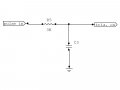Now we need to use logic thinking, as an engineer you will need to make choices on component values and such based on what your trying to achieve, this case we need to think of what kind of a ramp wave are we looking for, if we just throw any value capacitor in there, we will produce a ramp wave, but is it the ramp wave we require, isn't a ramp wave a ramp wave, sure, but what do you want this ramp wave to do. WE cannot just make a ramp wave network, and expect it to work for us, without a little planning ahead of time.

Lets determine what the ramp wave should do, this ramp wave is going to be used to turn on a switching circuit, we are going to use a analog signal (ramp wave) to produce a digital signal.
At this time you probably don't know what this ramp wave is going to do, but after you build this circuit, you should be able to know exactly what the ramp wave is used for.

So I am going to go through some basic details of what we want the ramp wave to look like.

1. we want it as large of voltage output as possible without distortion, (within reason)
2. we want the charge up time of the capacitor to be longer than the pulse driving it, so as to make sure we don't have clipping of the ramp wave on its positive peak. (the sharper the peak is the better control of driving the motor more accurately and precisely.)

Here is the first formula needed to determine the capacitor value. (C3).

TC = (R*C) means Time Constant:, this is the amount of time the capacitor will charge up to a voltage of around 63% of applied voltage. Engineers have established that it takes around 5 TC's to have a capacitor charge up to around 99% of applied voltage.

Now lets think carefully of what we want to do, we have a pulse of 2mS, coming into this network, we want the capacitor to charge up to a maximum voltage within that time frame, so since it is determined that (TC * 5) = full charge, then we will now substitute our requirements for a 2mS pulse which is (TC*5 = 2mS) which means in 2mS the cap. will get fully charged.

What was all of that about, it's this way, when the 2mS pulse comes into this network, that amount of pulse time, will fully charge up the capacitor. to a maximum voltage, according to the formula (5 * TC = cap. full charge)
So if five Time Constants fully charges the capacitor, then what value of capacitor do we need to achieve this.

We find that value by the equation (pw=5*TC) where pw is the pulse width which is the amount of time to charge the cap. to full charge, and TC is time constant, which is equal to (R*C).
so therefor (TC = pw / 5) so plugging in our values, (TC = 2mS / 5) which becomes (TC = 400uS)
So now we have determined one Time Constant is equal to 400uS. and now we use another equation to solve for the capacitor value, needed to give this result.
(TC = R*C) so now plugging in our values again, we have (400uS = 3K ohms * C) rearranging we have (C = 400uS / 3k ohms) = 133nF. pic.6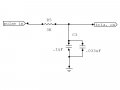now these are theoretical values, we may need to tweak the capacitor value as needed as we view the waveform on the oscilloscope.
lets see what the scope shows. pic7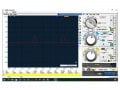As can be seen the top of the waveform has a little clipping this is because I used pw value in the equation to be equal with pulse coming in. Thats Ok because by using the values we used gets us in the ballpark, now is a good time to do some cap. value tweaking.
I'll make the cap. larger from 133nF to 200nF and see how the waveform looks. pic.8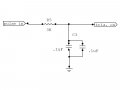To be continued on page 2 of 3 stay tuned.

#### MrChips

Joined Oct 2, 2009
28,137
The forum is not the place for this. This should go into a blog.

Status
Not open for further replies.